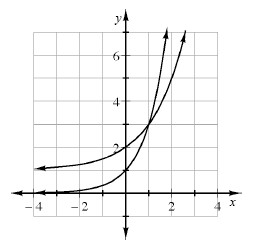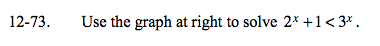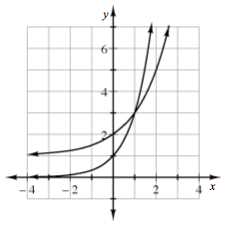### Home > A2C > Chapter 12 > Lesson 12.1.4 > Problem12-73

12-73.

Use the graph below to solve 2x + 1 < 3x . Homework Help ✎Since 3x has a higher multiplier, it is steeper than 2x + 1.

Where is the graph of 3x above the graph of 2x + 1?